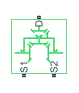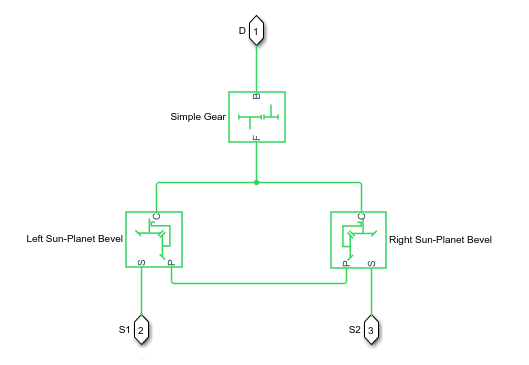# Differential

Gear mechanism that allows driven shafts to spin at different speeds

•Libraries:
Simscape / Driveline / Gears

## Description

The Differential block represents a gear mechanism that allows the driven shafts to spin at different speeds. Differentials are common in automobiles, where they enable the various wheels to spin at different speeds while cornering. Ports D, S1, and S2 represent the longitudinal driveshaft and the sun gear shafts of the differential, respectively. Any one of the shafts can drive the other two.

The block models the differential mechanism as a structural component based on the Simple Gear and Sun-Planet Bevel Simscape™ Driveline™ blocks. The figure demonstrates the equivalent block diagram for the Differential block.To increase the fidelity of the gear model, specify properties such as gear inertia, meshing losses, and viscous losses. By default, gear inertia and viscous losses are assumed to be negligible. The block enables you to specify the inertias of the gear carrier and internal planet gears. To model the inertias of the outer gears, connect Simscape Inertia blocks to ports D, S1, and S2.

### Thermal Modeling

You can model the effects of heat flow and temperature change by enabling the optional thermal port. To enable the port, set Friction model to ```Temperature-dependent efficiency```.

### Equations

Ideal Gear Constraints and Gear Ratios

The differential imposes one kinematic constraint on the three connected axes, such that

`${\omega }_{S1}-{\omega }_{S2},$`

where:

• ωS1 is the velocity of sun gear shaft 1.

• ωS2 is the velocity of sun gear shaft 2.

Negative values imply that the differential is left of centerline. The three degrees of freedom reduce to two independent degrees of freedom. The gear pairs are (1,2) = (S, S) and (C, D). C is the carrier.

The sum of the lateral motions is the transformed longitudinal motion. The difference of side motions, ${\omega }_{S1}-{\omega }_{S2}$, is independent of the longitudinal motion. The general motion of the lateral shafts is a superposition of these two independent degrees of freedom, which have this physical significance:

• The longitudinal degree of freedom is equivalent to the two lateral shafts rotating at the same angular velocity, ${\omega }_{S1}={\omega }_{S2}$, and at a fixed ratio with respect to the longitudinal shaft.

• The differential degree of freedom is equivalent to keeping the longitudinal driving shaft locked, ${\omega }_{D}=0$, where ωD is the velocity of the driving shaft, while the lateral shafts rotate with respect to each other in opposite directions, ${\omega }_{S1}=-{\omega }_{S2}$.

The lateral axis torques are constrained by the longitudinal axis torque such that the net power flow sums to zero:

`${\omega }_{S1}{\tau }_{S1}+{\omega }_{S2}{\tau }_{S2}+{\omega }_{D}{\tau }_{D}-{P}_{loss}=0,$`

where:

• τS1 and τS2 are the torques along the lateral axes.

• τD is the longitudinal torque.

• Ploss is the power loss.

When the kinematic and power constraints are combined, the ideal case yields

`${g}_{D}{\tau }_{D}=2\frac{\left({\omega }_{S1}{\tau }_{S1}+{\omega }_{S2}{\tau }_{S2}\right)}{{\omega }_{S1}+{\omega }_{S2}},$`

where gD is the gear ratio for the longitudinal driveshaft.

Ideal Fundamental Constraints

The effective Differential block constraint is composed of two sun-planet bevel gear subconstraints.

• The first subconstraint is due to the coupling of the two sun-planet bevel gears to the carrier:

`$\frac{{\omega }_{S1}-{\omega }_{C}}{{\omega }_{S2}-{\omega }_{C}}=-\frac{{g}_{SP2}}{{g}_{SP1}},$`

where gSP1 and gSP2 are the gear ratios for the sun-planet gears.

• The second subconstraint is due to the coupling of the carrier to the longitudinal driveshaft:

`${\omega }_{D}=-{g}_{D}{\omega }_{C}.$`

The sun-planet gear ratios of the underlying sun-planet bevel gears, in terms of the radii, r, of the sun-planet gears are:

`${g}_{SP1}=\frac{{r}_{S1}}{{r}_{P1}}$`

`${g}_{SP2}=\frac{{r}_{S2}}{{r}_{P2}}$`

The Differential block is implemented with ${g}_{SP1}={g}_{SP2}=1$, leaving gD free to adjust.

Nonideal Gear Constraints and Losses

In the nonideal case, τloss ≠ 0. For more information, see Model Gears with Losses.

### Assumptions and Limitations

• The gears are assumed to be rigid.

## Ports

### Conserving

expand all

Rotational mechanical conserving port associated with the longitudinal driveshaft.

Rotational conserving port associated with sun gear 1.

Rotational conserving port associated with sun gear 2.

Thermal conserving port associated with heat flow. Heat flow affects the power transmission efficiency by altering the gear temperatures.

#### Dependencies

To enable this port, set Friction model to `Temperature-dependent efficiency`.

## Parameters

expand all

### Main

Location of the bevel crown gear relative to the centerline of the gear assembly.

Fixed ratio, gD, of the carrier gear to the longitudinal driveshaft gear rotations as defined by the number of carrier gear teeth divided by the number of driveshaft gear teeth. This gear ratio must be strictly greater than `0`.

### Meshing Losses

Friction model for the block:

• `No meshing losses - Suitable for HIL simulation` — Gear meshing is ideal.

• `Constant efficiency` — Transfer of torque between the gear wheel pairs is reduced by a constant efficiency, η, such that 0 < η ≤ 1.

• `Temperature-dependent efficiency` — Transfer of torque between the gear wheel pairs is defined by the table lookup based on the temperature.

Vector of torque transfer efficiencies, [ηSS, ηCD], from driving to driven sun gear and from the carrier to the longitudinal driveshaft, respectively. The vector elements must be in the range (0,1].

#### Dependencies

To enable this parameter, set Friction model to `Constant efficiency`.

Vector of temperatures used to construct a 1-D temperature-efficiency lookup table. The vector elements must increase from left to right.

#### Dependencies

To enable this parameter, set Friction model to `Temperature-dependent efficiency`.

Vector of output-to-input power ratios that describe the power flow from the driving sun gear to the driven sun gear, ηSS. The block uses the values to construct a 1-D temperature-efficiency lookup table.

Each element is an efficiency that relates to a temperature in the Temperature vector. The length of the vector must be equal to the length of the Temperature vector. Each element in the vector must be in the range (0,1].

#### Dependencies

To enable this parameter, set Friction model to ```Temperature-dependent efficiency```.

Vector of output-to-input power ratios that describe the power flow from the carrier to the driveshaft, ηCD. The block uses the values to construct a 1-D temperature-efficiency lookup table.

Each element is an efficiency that relates to a temperature in the Temperature vector. The length of the vector must be equal to the length of the Temperature vector. Each element in the vector must be in the range (0,1].

#### Dependencies

To enable this parameter, set Friction model to ```Temperature-dependent efficiency```.

Vector of power thresholds, pth, for sun-carrier and longitudinal driveshaft-casing [pS, pD], respectively. The full efficiency loss applies above these values. Below these values, a hyperbolic tangent function smooths the efficiency factor.

When you set Friction model to `Constant efficiency`, the block lowers the efficiency losses to zero when no power is transmitted. When you set Friction model to `Temperature-dependent efficiency`, the block smooths the efficiency factors between zero when at rest and the values provided by the temperature-efficiency lookup tables at the power thresholds.

#### Dependencies

To enable this parameter, set Friction model to `Constant efficiency` or ```Temperature-dependent efficiency```.

### Viscous Losses

Vector of viscous friction coefficients, [μS μD ], for the sun gear-to-carrier gears and longitudinal driveshaft-to-casing gear motions, respectively.

### Inertia

Inertia model for the block:

• `Off` — Model gear inertia.

• `On` — Neglect gear inertia.

Moment of inertia of the planet gear carrier. This value must be positive.

#### Dependencies

To enable this parameter, set Inertia to `On`.

Moment of inertia of the combined planet gears. This value must be positive.

#### Dependencies

To enable this parameter, set Inertia to `On`.

### Thermal Port

To enable these settings, set Friction model to `Temperature-dependent efficiency`.

Thermal energy required to change the component temperature by a single temperature unit. The greater the thermal mass, the more resistant the component is to temperature change.

#### Dependencies

To enable this parameter, set Friction model to ```Temperature-dependent efficiency```.

Block temperature at the start of simulation. The initial temperature sets the initial component efficiencies according to their respective efficiency vectors.

#### Dependencies

To enable this parameter, set Friction model to `Temperature-dependent efficiency`.